JAJSIU8B June   2015  – April 2020

PRODUCTION DATA.

1. 特長
2. アプリケーション
3. 説明
4. 改訂履歴
5. Device Comparison
6. Pin Configuration and Functions
7. Specifications
8. Parameter Measurement Information
9. Detailed Description
1. 9.1 Overview
2. 9.2 Functional Block Diagram
3. 9.3 Feature Description
4. 9.4 Device Functional Modes
1. 9.4.1 Power-Down
2. 9.4.2 Reset
3. 9.4.3 Conversion Mode
5. 9.5 Programming
1. 9.5.1 SPI Interface
2. 9.5.2 Data Retrieval
3. 9.5.3 SPI Command Definitions
6. 9.6 Register Map
1. 9.6.1 Register Descriptions
10. 10Application and Implementation
1. 10.1 Application Information
2. 10.2 Typical Application
3. 10.3 Initialization Set Up
11. 11Power Supply Recommendations
12. 12Layout
13. 13デバイスおよびドキュメントのサポート
1. 13.1 ドキュメントのサポート
2. 13.2 サポート・リソース
3. 13.3 商標
4. 13.4 静電気放電に関する注意事項
5. 13.5 Glossary
14. 14メカニカル、パッケージ、および注文情報

• PAG|64
• PAG|64

### 7.8 Typical Characteristics

at TA = 25°C, AVDD = 3 V, AVSS = 0 V, DVDD = 1.8 V, internal VREFP = 2.4 V, VREFN = AVSS, external fCLK = 2.048 MHz, data rate = 8 kSPS, and gain = 1 (unless otherwise noted)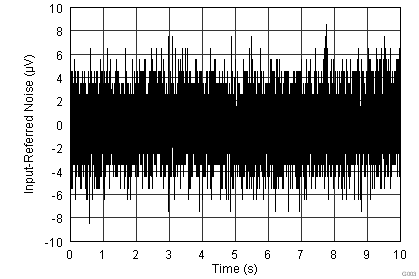Data rate = 1 kSPS, gain = 1
Figure 3. Input-Referred Noise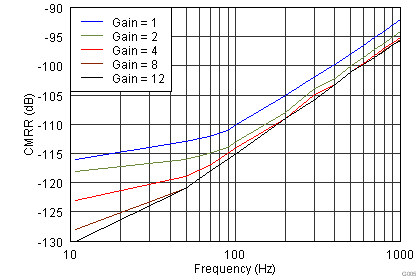Data rate = 4 kSPS, VIN = AVDD – 0.3 V to AVSS + 0.3 V
Figure 5. Common-Mode Rejection Ratio vs Frequency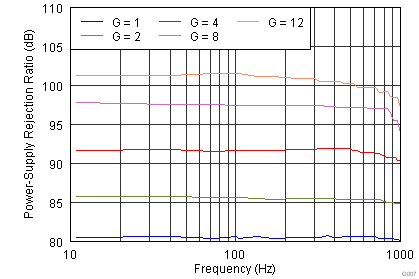Figure 7. PSRR vs Frequency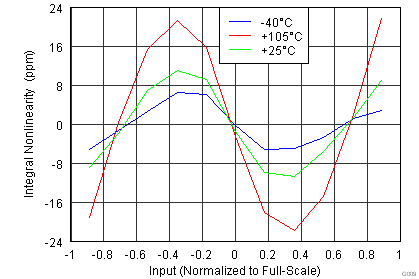Figure 9. INL vs Temperature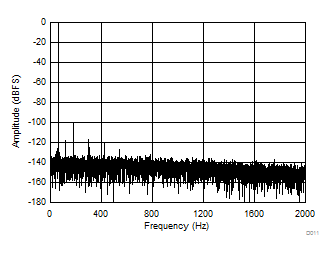Gain = 1, THD = –100 dB, SNR = 107 dB, data rate = 4 kSPS, 16384 points
Figure 11. THD FFT Plot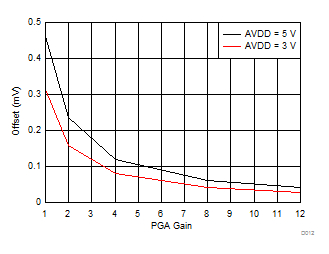Figure 13. Offset vs PGA Gain (Absolute Value)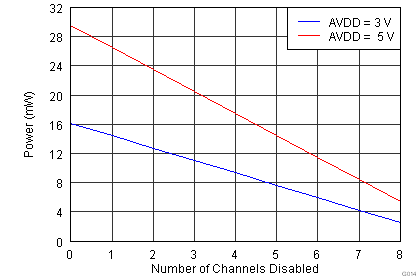Figure 15. Channel PowerData rate = 1 kSPS, gain = 1
Figure 4. Noise Histogram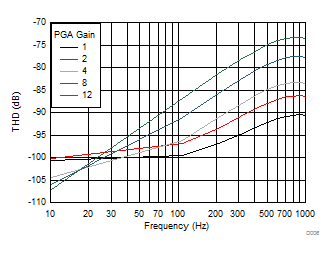Figure 6. Total Harmonic Distortion vs Frequency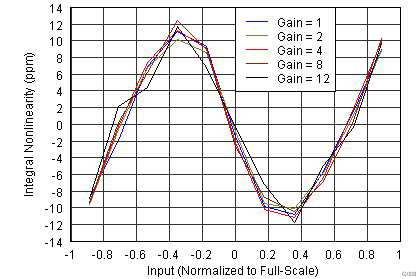Figure 8. INL vs PGA Gain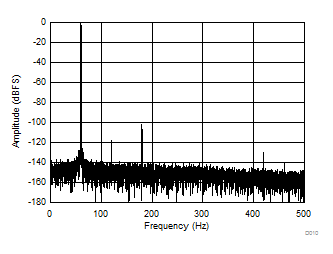Gain = 1, THD = –101.5 dB, SNR = 111 dB, data rate = 1 kSPS, 16384 points
Figure 10. THD FFT PlotGain = 1, THD = –100 dB, SNR = 76 dB, data rate = 64 kSPS, 16384 points
Figure 12. FFT Plot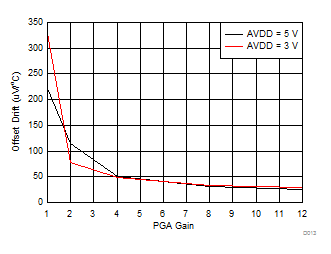Figure 14. Offset Drift vs PGA Gain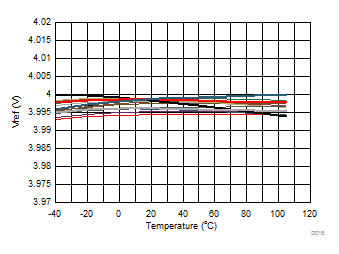AVDD = 5 V, AVSS = 0 V, VREF = 4 V
Figure 16. Internal VREF vs Temperature